Chapter 17: Device driver, Local variables, and LCD output
Modified to be compatible with EE319K Lab 7

Embedded Systems - Shape The World
Jonathan Valvano and Ramesh Yerraballi

Learning Objectives:

• Variables
• Discuss scope and allocation
• Present techniques to implement local variables on the stack
• Stack frames
• I/O Device driver
• Hardware interface to the ST7735R
• A set of software functions
• Synchronization
• Define fixed-point numbers.
• Present methods to display numbers on the LCD
• Introduce recursion as a software design technique.

Video 17. Introduction to Chapter 17, and EE319K Lab 7.

## 17.1. Variables

Video 17. Local versus global variables.

Program 17.1 shows the main.c file used in the video. Program 17.2 shows the Logger.c file. Click this link to download entire project.

// main.c
#include "UART.h"
#include "random.h"
#include "Logger.h"
// Global Variables in RAM
char *progtitle="Histogram of Randoms"; // Global Scope and
// Permanent persistence (RAM)
// The entry point is a function with global scope
int main(){
uint32_t i; // Local scope (in main) and persists
// as long as main does does not return
// allocated on the Stack
Output_Init();
Random_Init(1317); // Initialize the Random Number Generator
for (i=0; i < 100; i++){
uint32_t val; // Local scope (for loop), and persists
// while the for loop runs
// allocated on the Stack
val = Random();
Logger_track(val%MAXVAL);
}
Logger_display();
while(1);
}

Program 17.1. The main.c file used in the above video.

// Logger.c
// Keeps track of the frequencies of values in a local array
// and displays them like a histogram when requested
#include
#include "Logger.h"
#define LineWidth 40
static uint8_t Frequency[MAXVAL]; // Local scope (to file Logger.c)
// permanent persistence (RAM)
extern char *progtitle;
static void pretty_print(uint8_t, uint8_t); //Prototype
uint8_t i; // Local scope (in LogInit) and persists
// as long as LogInit does does not return;
// allocated on the Stack
for (i=0; i < MAXVAL; i++)
Frequency[i]=0;
}
// Keeps track of values on successive calls
// in the Log array
uint8_t Logger_track(uint32_t val){
static uint8_t first=0; // Local (in Logger_track)
// permanent persistence (RAM)
if(first == 0){
first=1;
}
if(val > MAXVAL) return(0); // Error check - fail
Frequency[val]++; //Increment frequency of the value
return(1); //success
}
void Logger_display(){
uint8_t index;
printf("%s\n",progtitle);
for (index=0; index< MAXVAL; index++){
pretty_print(index,Frequency[index]);
}
}

// Local (to file) static function that can only be called from
// within this file
static void pretty_print(uint8_t val, uint8_t times){
uint8_t i;
printf("%d:",val);
for(i=0; i < times; i++){
if (i >= LineWidth) break;
printf("*");
}
printf("%d\n",times);
}

Program 17.2. The Logger.c file used in the above video.

Variables are an important component of software design, and there are many factors to consider when creating variables. Some of the obvious considerations are the size and format of the data. In this class we will consider integers, which can be 8-bit, 16-bit or 32 bits. Furthermore, integers can signed or unsigned. The following table shows the C99 type definitions,

PrecisionUnsignedSigned
8 bits uint8_t int8_t
16 bits uint16_t int16_t
32 bits uint32_t int32_t

Table 17.1. C99 type definitions for integers.

Another factor is the scope of a variable. The scope of a variable defines which software modules can access the data. Variables with an access that is restricted to one software module are classified as private, and variables shared between multiple modules are public. In general, a system is easier to design (because the modules are smaller and simpler), easier to change (because code can be reused), and easier to verify (because interactions between modules are well-defined) when we limit the scope of our variables. However, since modules are not completely independent we need a mechanism to transfer information from one to another. The ARM Application Binary Interface (ABI) has detailed descriptions of how to develop software interfaces. However, in this chapter, we will discuss the fundamentals of software interfaces.

An addition consideration for variables is allocation or persistance. We could place variables in registers temporarily, on the stack in RAM temporarily, in RAM permanently, or in ROM permanently. We will use the terms allocated permanently and permanent persistence to mean the same thing, created at compile time and never destroyed. Because their contents are allowed to change, all variables must be allocated in registers or RAM and not ROM. Constants can be placed in ROM. A local variable has reduced scope and temporary allocation. We can allocate a local variable in a register or on the stack. One of the important objectives of this chapter is to present design steps for creating, using, and destroying local variables on the stack. In C, we create a local variable by defining it within the function. We will consider parameters passed into or out of a function as local variables, because they have reduced scope and temporary allocation. The scope of the variable `sum` is within the entire function, whereas the scope of `i` is within the for-loop. Local variables are not initialized. Therefore it is your responsibility to initialize your local varaibles.

uint32_t MyFunction(void){uint32_t sum;
sum = 0;
for(uint32_t i=0; i < 10; i++){
sum=sum+i;
}
return sum;
}

A static variable has reduced scope and permanent persistence. We allocate static variables in permanent RAM. The scope can be reduced to a single function or a single file. Static variables will be initialized to 0 on software reset, or we can explicitly initialize it. It is good programming practice to initialize all your varaibles, even if the compiler does initialize them to 0. Static variables are initialized just once, at reset. In this example `TotalCount` is initialized once to 0, it is shared within the file, so accessible to both functions. `TotalCount` contains the total number of times either function has been called. There are two copies of `Num`, one for each function. The static `Num` variable maintains the number of times each function has been called. The two functions will return 1 if that function has been called more than 75 times or if the sum of the two calls is more than 100.

static uint32_t TotalCount=0;
uint32_t MyFunction2(void){
static uint32_t Num=0;
Num++; TotalCount++;
if((Num > 75)||(Count > 100)){
return 1;   }
return 0;
}
uint32_t MyFunction3(void){
static uint32_t Num=0;
Num++; TotalCount++;
if((Num > 75)||(Count > 100)){
return 1;   }
return 0;
}

A global variable has public scope and permanent persistence. Public scope means any software in the system has access to the variable. Global variables are permanently allocated in RAM. Global variables will be initialized to 0 on software reset, unless we can explicitly initialize it to something else. We will consider I/O port registers as global variables, because they have public scope and permanent persistence. The global variable `Flag` can be accessed by both `MyFunction4` and `MyFunction5`, even if the functions are in different files. The `extern` definition does not create a second copy of the variable, rather, it provides access to the single shared global.

// Assume Flag and MyFunction 4 are in one file
uint32_t Flag;
void MyFunction4(void){
Flag = 0;
}
// Assume MyFunction 5 is in a different file
extern uint32_t Flag;
void MyFunction5(void){
Flag = 1;
}

In general, the qualifier const added to a variable definition means the software cannot change its value. In embedded systems with RAM and ROM, const added to a global variable means it will be allocated in ROM permanently (permanent persistence). The global constant `Size` can be accessed anywhere in the software system, but cannot be dynamically changed.

const uint32_t Size=100;
void MyFunction6(void){
for(uint32_t i=0; i < Size; i++){
// stuff
}
}

When the qualifier const added to a parameter it means the software cannot change its value within the function. The parameter `Size` can be accessed in the function, but cannot be dynamically changed. In this example, the parameter `Size` is still passed in Register R0, with temporary allocation and private scope.

void MyFunction7(const uint32_t Size){
for(uint32_t i=0; i < Size; i++){
// stuff
}
}

A static function has reduced scope. On an embedded system, all functions are permanentally allocated in ROM. If we add static to a function definition, the scope can be reduced to file in which it is defined. This means only functions also defined in this file can call it. Other names for reduced scope functions are private functions and helper functions. In general, it is good design to reduce scope of data and functions as much as possible. Prototypes for public functions are placed in the header file, whereas prototypes for static functions are not placed in the header file. This way we can separate what a module does (by calling public functions) from how it works (implementation of all functions including static functions). In the following example, the function `rand` is static, so it is callable within the file. On the other hand, the function `Random` is public and can be called from anywhere.

uint32_t static M=1;
uint32_t static rand(void){
M = 1664525*M+1013904223;
return(M);
}
uint8_t Random(void){
return(rand()>>24);
}

: How do you create a local variable in C?

: How do you create a global variable in C?

: Considering scope and allocation, what changes and what doesn't change when you add static to an otherwise global variable?

: Considering scope and allocation, what doesn't change when you add static to an otherwise local variable?

: Considering scope and allocation, what changes and what doesn't change when you add const to an otherwise global variable?

: Considering scope and allocation, what changes and what doesn't change when you add const to a function parameter?

The following video presents the implementation of local variables on the stack using SP-relative addressing

Video 17. Locals in assembly.

Program 17.3 shows the sum.c file used in the video. Program 17.4 shows the main.s file. Click this link to download entire project.

//------------Sum------------
// Input: num is a 32-bit unsigned int
// Output: Is the sum: 1+2+...+num
// Here is the C code
uint32_t Sum (uint32_t num){
uint32_t i, result=0;
for (i=1; i <= num; i++){
result += i;
}
return(result);
}

Program 17.3. The sum.c file used in the above video.

EXPORT Start
Start
; Call the non-recursive implementation with locals on stack
MOV R0, #10
BL Sum ; R0 must be 55: 1+2+3...+10
Loop B Loop ; Loop forever

;------------Sum------------
; Input: R0 has input number (num)
; Output: R0 has the output which is the sum: 1+2+...+num
; Here is the Assembly Code
i equ 0 ; *Binding*: Local variable i is at offset 0 w.r.t SP
result equ 4 ; Local variable result is at offset 0 w.r.t SP
Sum
PUSH {R4,R5,LR} ; push things we will use for scratch
SUB SP,#8 ; *Allocation*: Allocate space for
; 2 local variables both 32-bit
MOV R4, #0
STR R4,[SP,#result] ; *Access* Initialize Result on stack
MOV R4, #1
STR R4,[SP,#i] ; *Access* Initialize index i on stack
LoopS
LDR R4,[SP,#i] ; *Access* load i into R4 from Stack
CMP R4,R0
BHI DoneS
LDR R5,[SP,#result] ; *Access* load result into R5 from Stack
ADD R5,R4 ; Result = Result + i;
STR R5,[SP,#result] ; *Access* store result from R5 to Stack
STR R4,[SP,#i] ; *Access* store i from R5 to Stack
B LoopS
DoneS
LDR R0,[SP,#result] ; *Access* load Result in R0 from Stack
ADD SP,#8 ; *DeAllocation* Deallocate space for locals
POP {R4,R5,PC} ; Restore scratched registers and set pushed
; LR to PC to return

Program 17.4. The main.s file used in the above video.

Video 17. Debugging Locals in assembly.

The following assembly code shows the PUSH and POP instructions can be used to store temporary information on the stack. If a subroutine modifies a register, it is a matter of programmer style as to whether or not it should save and restore the register. According to AAPCS a subroutine can freely change R0–R3 and R12, but the subroutine must save and restore any other register it changes. In particular, if one subroutine calls another subroutine, then it must save and restore the LR. In the following example, assume the function modifies Register R0, R4, R8 and calls another function. The programming style dictates registers R4, R8, and LR be saved. Notice the return address is pushed on the stack as LR but popped off into PC. When multiple registers are pushed or popped, the data exist in memory with the lowest numbered register using the lowest memory address. In other words, the registers in the {} can be specified in any order, but the order in which they appear on the stack is fixed. According to AAPCS we must push and pop an even number of registers. Of course remember to balance the stack by having the same number of pops as pushes.

Func PUSH {R4,R5,R8,LR} ; save registers as needed
;1) allocate local variables
;2) body of the function, access local variables
;3) deallocate local variables
POP {R4,R5,R8,PC} ; restore registers and return

The ARM processor has a lot of registers, and we appropriately should use them for temporary information such as function parameters and local variables. However, when there are a lot of parameters or local variables, we can place them on the stack. Program 17.5 has a large data buffer that is private to this function. It is inconvenient to store arrays in registers. Rather it is appropriate to place the array in memory and use indexed addressing mode to access the information. Because this buffer is private and temporary we will place it on the stack. 1) The SUB instruction allocates 10 words on the stack. Figure 17.1 shows the stack before and after the allocation. 2) During the execution of the function, the SP points to the first location of data. The local variable i is held in R0. R1 will contain i*4 as an offset into the buffer, because each buffer entry is 4 bytes. The addressing mode [SP,R1] accesses data on the stack without pushing or popping. 3) The ADD instruction deallocates the local variable, balancing the stack.

 Set  SUB SP,SP,#40  ;1)allocate 10 words          MOVS R0,#0x00  ;2)i=0      B test         ;2) loop LSL R1,R0,#2   ;2)4*i      STR R0,[SP,R1] ;2)access      ADDS R0,R0,#1  ;2)i++ test CMP R0,#10     ;2)      BLT loop       ;2)      ADD SP,SP,#40  ;3)deallocate      BX LR // C language implementation void Set(void){ uint32_t data; int i;   for(i=0; i<10; i++){     data[i] = i;   } }

Program 17.5. Allocation of a local array on the stack.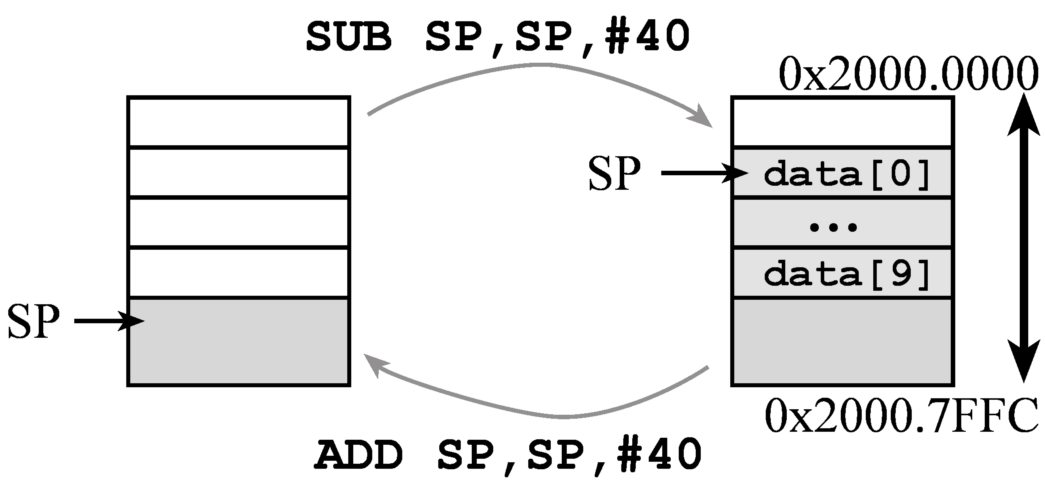Figure 17.1. Allocation of a local array on the stack.

Stack implementation of local variables has four stages: binding, allocation, access, and deallocation. In this section, the software will create two local variables called sum and i.

1. Binding is the assignment of the address (not value) to a symbolic name. In other words, we assign offsets for the variables. In general, we perform binding by drawing a stack picture and deciding the order of the local variables, see Figure 17.2. The symbolic name will be used by the programmer when referring to the local variable. The assembler binds the symbolic name to a stack index, and the computer calculates the physical location during execution. In the following example, the local variable sum will be at address SP+0, and the programmer will access the variable using [SP,#sum] addressing. Similarly, the local variable i will be at address SP+4, and the programmer will access the variable using [SP,#i] addressing:

sum EQU 0  ;32-bit local variable, stored on the stack
i   EQU 4  ;32-bit local variable, stored on the stack

2. Allocation is the generation of memory storage for the local variable, or assigning space. The computer allocates space during execution by decrementing the SP. In this first example, the software allocates the local variable by pushing a register on the stack. The variable sum is initialized to 0 and the variable i is initialized to 16. According to AAPCS, we must allocate space in multiples of 8 bytes. The contents of the register become the initial value of the variable.

MOV R0,#0
MOV R1,#16
PUSH {R0,R1}  ;allocate and initialize two 32-bit variables

Rather than creating local variables with initialization, the software could allocate the local variables by decrementing the stack pointer. Allocating locals this way creates them uninitialized. This method is most general, allowing the allocation of an arbitrary amount of data.

SUB SP,#8  ;allocate two 32-bit variables

3. The access to a local variable is a read or write operation that occurs during execution. Because we use SP addressing with offset, we will only use LDR and STR to access local variables on the stack. In the first code fragment, we will add the contents of i to the local variable sum.

LDR R0,[SP,#i]    ; R0=i
LDR R1,[SP,#sum]  ; R1=sum
STR R1,[SP,#sum]  ; sum=i+sum

In the next code fragment, the local variable sum is divided by 16.

LDR R0,[SP,#sum]  ; R0=sum
LSR R0,R0,#4
STR R0,[SP,#sum]  ; sum=sum/16

4. Deallocation is the release of memory storage for the location variable. This step frees up space. The computer deallocates space during execution by incrementing SP. The software deallocates two local variables by incrementing the stack pointer. When deallocating, we must balance the stack. I.e., we add to the SP exactly the same number as we decremented during allocation.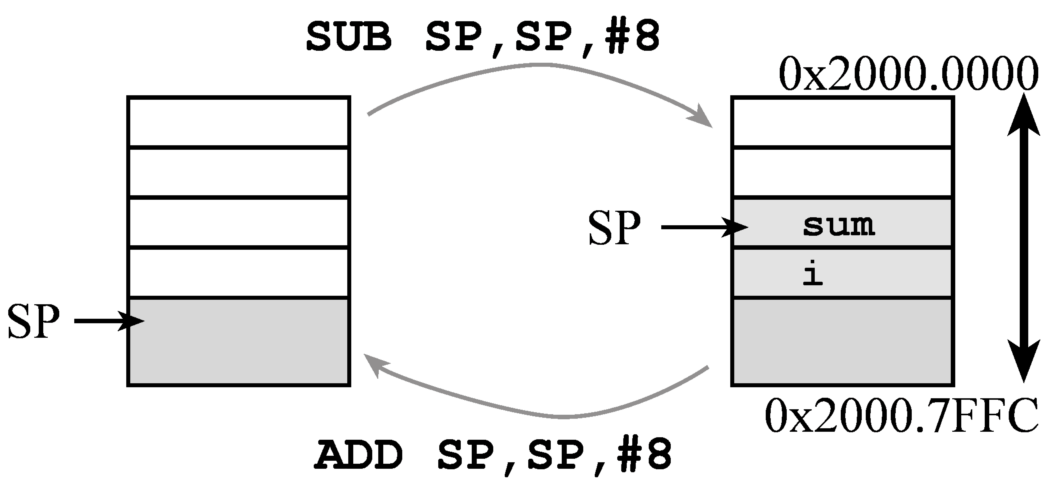Figure 17.2. Allocation of two local variables on the stack.

Program 17.6 shows a C and assembly function implementing the same function. This assembly implementation uses the PUSH instruction to allocate and initialize the local variables.

 Calculate   MOV R0,#0   MOV R1,#16   PUSH {R0,R1}  ;allocate and initialize     loop   LDR R0,[SP,#i]    ; R0=i   LDR R1,[SP,#sum]  ; R1=sum   ADD R1,R0         ; R1=i+sum   STR R1,[SP,#sum]  ; sum=i+sum   LDR R0,[SP,#i]    ; R0=i   SUBS R0,R0,#1     ; R0=i-1   STR R0,[SP,#i]   BNE loop   LDR R0,[SP,#sum]  ; R0=sum   LSR R0,R0,#4   STR R0,[SP,#sum]  ; sum=sum/16   ADD SP,SP,#8      ; deallocate   BX LR // C language implementation uint32_t Calculate(void){ uint32_t sum=0; uint32_t i=16;   do{     sum = i+sum;     i = i-1;   }   while(i != 0)   sum = sum/16;   return sum; }

Program 17.6. Allocation of two local variables on the stack.

: Write code that allocates four 32-bit local variables, uninitialized.

: Write code that binds four 32-bit local variables to the names a,b,c,d such that a is on top.

: Assuming the name of a 32-bit local variable is b, write code that sets b to 5.

: Write code that deallocates four 32-bit local variables.

: Assume Register R0 contains the size in 32-bit words of an array, determined at run-time. Write assembly code to allocate the array on the stack.

## Stack frames

Each time a function is called a stack frame is created. There are four types of data that may be saved in the stack frame. By convention, if there are more than 4 input parameters, additional parameters above 4 will be pushed on the stack by the calling program. If the function calls another function, the LR (return address) must be pushed on the stack. By convention if the function uses registers R4–R11, it will push them on the stack so their values are preserved. Lastly, the function may allocate local variables on the stack.

Video 17. Local variables using a stack frame.

Each time a function is called a stack frame is created. There are four types of data that may be saved in the stack frame. By convention, if there are more than 4 input parameters, additional parameters above 4 will be pushed on the stack by the calling program. If the function calls another function, the LR (return address) must be pushed on the stack. By convention if the function uses registers R4–R11, it will push them on the stack so their values are preserved. Lastly, the function may allocate local variables on the stack.

One limitation of SP indexed addressing mode to access local variables is the difficulty of pushing additional data onto the stack during the execution of the function. In particular, if the body of the function pushes additional items on the stack, the symbolic binding becomes incorrect. There are two approaches to this problem. First, we could recompute the binding after each stack push/pop. Second, we could assign a second register to point into the stack. To employ a stack frame pointer we execute the initial steps of the function: saving LR, saving registers, and allocating local variables on the stack. Once these initial steps are complete, we set another register to point into the stack. Because R4–R11 will be saved and restored any of these would be appropriate for the stack frame pointer. E.g.,

MOV R11,SP

This stack frame pointer (R11) points to the local variables and parameters of the function. It is important in this implementation that once the stack frame pointer is established (e.g., using the MOV R11,SP instruction), that the stack frame register (R11) not be modified. The term frame refers to the fact that the pointer value is fixed. If R11 is a fixed pointer to the set of local variables, then a fixed binding (using the EQU pseudo op) can be established between Register R11 and the local variables and parameters, even if additional information is pushed on the stack. Because the stack frame pointer should not be modified, every subroutine will save the old stack frame pointer of the function that called the subroutine and restore it before returning. Local variable access uses indexed addressing mode using Register R11.

: When should we use stack frames with R11 addressing instead of regular local variables with SP addressing?

: When implementing stack frames with R11 addressing, do we subtract from R11 or from SP when allocating local variables?

One of the advantages of ARM Architecture Procedure Call Standard (AAPCS) is that we can write one function in one environment (C or assembly) and invoke it from another environment. Recall the rules of AAPCS:

• Pass input parameters in R0, R1, R2, R3 as needed.
• Return output parameter in R0 if needed.
• The function can freely use R0,R1,R2,R3,R12.
• The function can use R4–R11, but save them at beginning and restore them at the end.
• Push and pop even numbers of registers

To better understand this video, open the Lab 7 starter project.

Video 17. Linking C to assembly.

In the following example, Program 17.7, the C function on the left calls an assembly function on the right. C needs a function prototype. In Lab 7, you will see we put function prototypes in a separate print.h header file. However in this example, the prototype is simply placed above the C program. In the assembly file, we specify the assembly function as public by exporting its address.

 // C program that invokes // an assembly function uint32_t sqrt(uint32_t s); // prototype     uint32_t HighLevel(void){   uint32_t input;   uint32_t output;   input=ReadInput();   output=sqrt(input); // invoke   return output; } ; low level assembly     EXPORT sqrt ;Input: R0 unsigned integer ;Output: R0 squareroot of input sqrt MOV  r1,r0      MOVS r3,#0x01      ADD  r0,r3,r1,LSR #4      MOVS r2,#0x10 loop MLA  r3,r0,r0,r1      UDIV r3,r3,r0      LSRS r0,r3,#1      SUBS r2,r2,#1      CMP  r2,#0x00      BNE  loop      BX   lr

Program 17.7. C program calls an assembly function.

In this next example, Program 17.8, the assembly function on the left calls a C function on the right. There is no need for a prototype for an assembly language to call a C function; both do need to follow AAPCS. The C compiler automatically creates AAPCS-compliant code. To link the C function into the assembly file, we use the IMPORT pseudo-op inside the assembly file. In the C file, we simply define the function.

 ; Assembly program that invokes    ;  a C function        IMPORT sqrt HighLevel     PUSH {R4,LR}     BL   ReadInput ; R0 has input parameter     BL   sqrt   ; invoke    ; R0 has output parameter     POP  {R4,PC} // low level C uint32_t sqrt(uint32_t s){ uint32_t t; // t*t will become s int n; // loop counter   t = s/16+1; // initial guess   for(n = 16; n; --n){     t = ((t*t+s)/t)/2;   }   return t; }

Program 17.8. C program calls an assembly function.

## 17.2. ST7735R Interfacing

In this section we will interface a ST7735R LCD using busy-wait synchronization. See Figures 17.3 and 17.4. Before we output data or commands to the display, we will check a status flag and wait for the previous operation to complete. Busy-wait synchronization is very simple and is appropriate for I/O devices that are fast and predicable.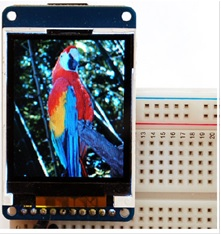Figure 17.3. ST7735R display with 160 by 128 16-bit color pixels.

Video 17. Interfacing the ST7735R LCD.

: How does the ST7735R software driver specify color?

D/C stands for data/command; you will make D/C high to send data and low to send a command. Because the LCD is so fast we will use "busy-wait" synchronization, which means before the software issues an output command to the LCD, it will wait until the display is not busy. In particular, the software will wait for the previous LCD command to complete.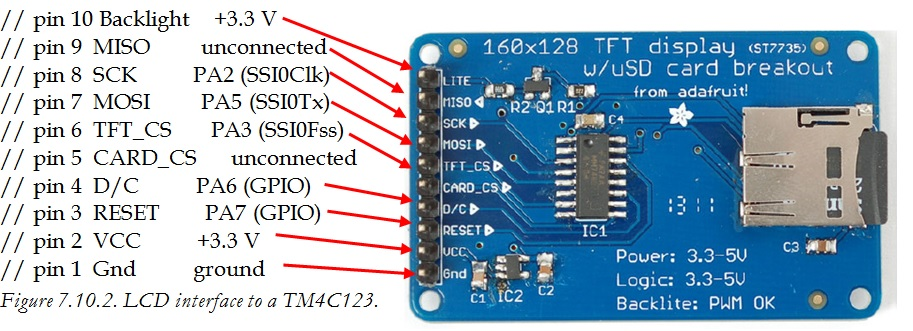Figure 17.4. LCD interface to a TM4C123.

: What does the D/C pin do?

: What does the TFT_CS pin do?

: What does the MOSI pin do?

: What does the SCK pin do?

Video 17. Synchronizing software to hardware.

The following pseudo-code and Figure 17.5 shows the steps to interact with the LCD using the SPI module. The SPI module uses a first in first out (FIFO) queue built into the hardware. Bit 4 of the SSI0_SR_R register is busy. If busy is 1, it means it cannot accept another command at this point. If busy is 0, it means it ready and can accept another command. Bit 1 of the SSI0_SR_R register is TNF, which stands for transmitter FIFO not full. If TNF is 0, it means the transmitter FIFO is full and it cannot accept another data output at this point. If TNF is 1, it means the FIFO is not full and can accept another data output. Notice that this interface will wait before and after each command, however multiple data outputs can occur as long as there in room in the FIFO.

writecommand: Involves 6 steps performed to send 8-bit Commands to the LCD
1. Read SSI0_SR_R and check bit 4,
2. If bit 4 is high, loop back to step 1 (wait for BUSY bit to be low)
3. Clear D/C=PA6 to zero (D/C pin configured for COMMAND)
4. Write the command to SSI0_DR_R
5. Read SSI0_SR_R and check bit 4,
6. If bit 4 is high loop back to step 5 (wait for BUSY bit to be low)

writedata: Involves 4 steps performed to send 8-bit Data to the LCD:
1. Read SSI0_SR_R and check bit 1,
2. If bit 1 is low, loop back to step 1 (wait for TNF bit to be one)
3. Set D/C=PA6 to one (D/C pin configured for DATA)
4. Write the 8-bit data to SSI0_DR_R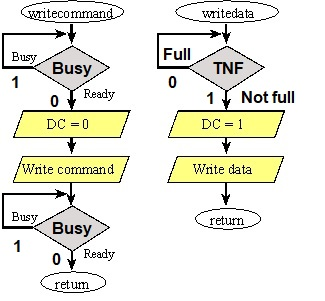Figure 17.5. Busy-wait synchronization is used to send commands and data to the display.

: What does busy-wait mean?

At the lowest level each ASCII character is mapped to an image. This mapping is called a font. The following figure and program shows how the character '6' is created on the screen as a 5 by 8 pixel image. The driver automatically inserts one blank line in between characters, so each character requires 6 by 8 pixels on the screen.Figure 17.6. ST7735R character font is 5 wide by 8-tall pixels.

static const uint8_t Font[] = {
0x00, 0x00, 0x00, 0x00, 0x00, // 0x00
0x3E, 0x5B, 0x4F, 0x5B, 0x3E, // 0x01
...
0x3C, 0x4A, 0x49, 0x49, 0x31, // 0x36= '6'
...
0x00, 0x00, 0x00, 0x00, 0x00 // 0xFF
};

Program 17.9. ST7735R character font is 5 wide by 8-tall pixels.

There is one image for all 8 bit possibilities from 0 to 0xFF. To handle extended ASCII, which are the values 0x80 to 0xFF, make sure to change the compiler settings to select unsigned for the char type. Execute Project->Options, in the C/C++ tab deselect the box "Plain char is signed", making char unsigned.

: How many characters can fit across one row of the LCD screen?

: The ST7735R software driver uses 10 pixels in the vertical direction for each row of characters. How many rows of characters can fit on the LCD screen?

There is a rich set of graphics functions available for the ST7735R, allowing you to create amplitude versus time, or bit-mapped graphics. Refer to the ST7735R.h header file for more details.

## 17.3. Nokia 5110 Interfacing

In this section we will interface a Nokia 5110 LCD using busy-wait synchronization. See Figures 17.xx and 17.xx. Before we output data or commands to the display, we will check a status flag and wait for the previous operation to complete. Busy-wait synchronization is very simple and is appropriate for I/O devices that are fast and predicable.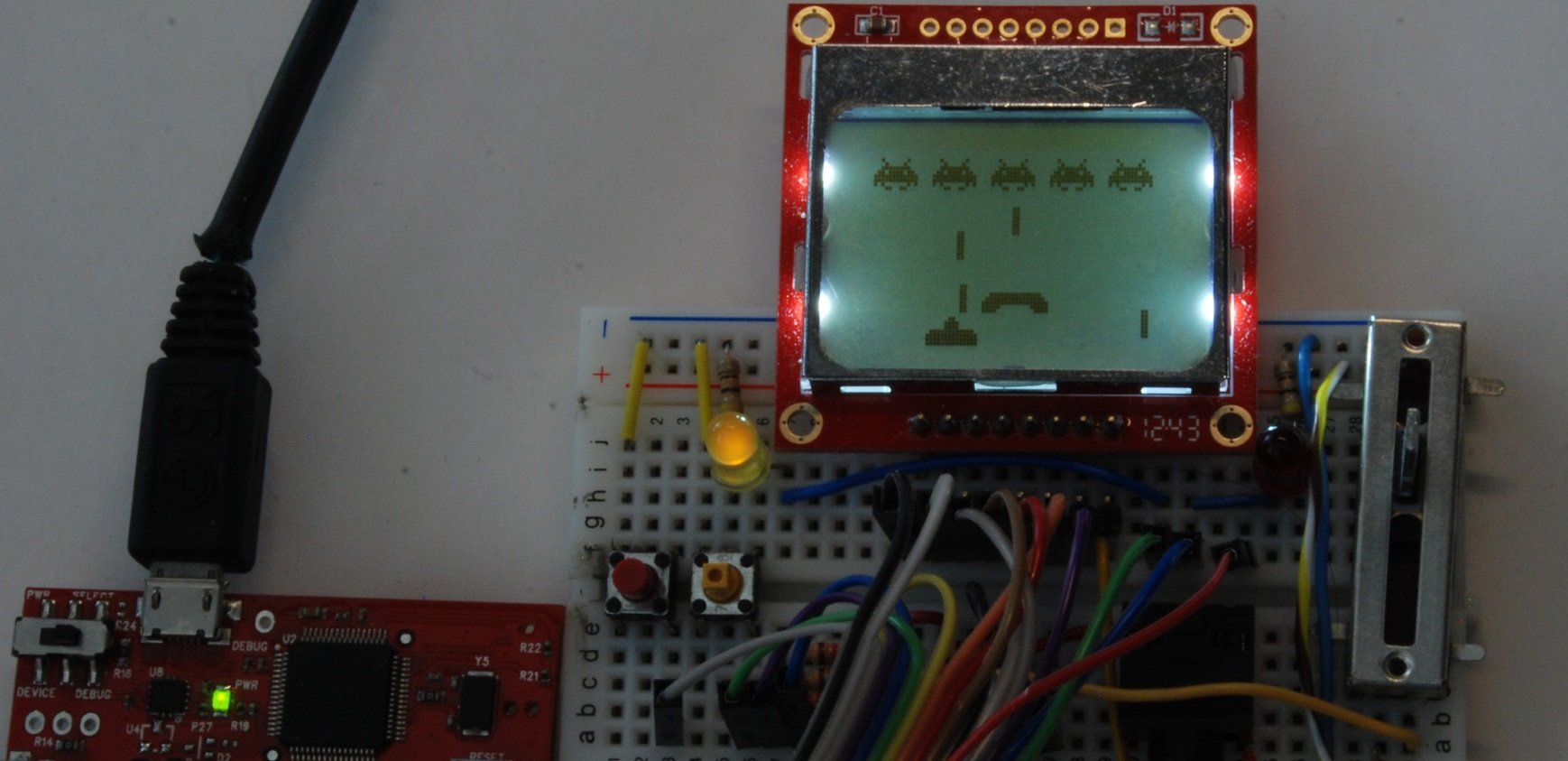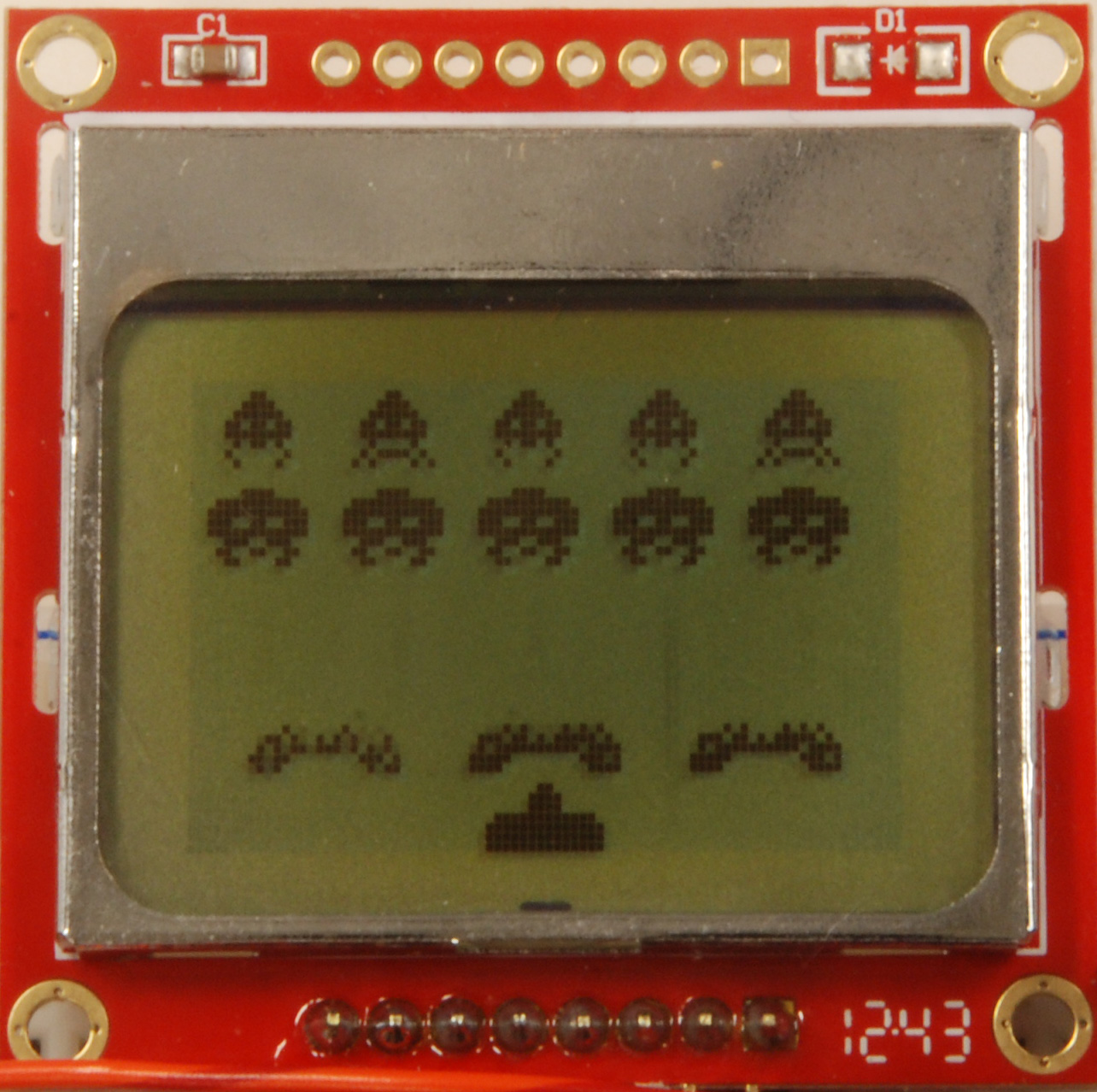Figure 17.7. Nokia 5110 display with 84 by 48 monochrome pixels.

The Nokia 5110 uses the synchronous serial interface (SSI) described in the last section to control PA5 (MOSI), PA3 (Fss), and PA2 (Sclk), as shown in Figure 8.14. Pins PA7 and PA6 are regular GPIO pins. The microcontroller will be master and the LCD slave. There are multiple Nokia 5110 displays for sale on the market with the same LCD but different pin locations for the signals. Figure 8.14 shows two of the possible pin configurations. Please look on your actual display for the pin name and not the pin number. The back light is optional. Be careful when connecting the backlight, because at 3.3V the back light draws 80 mA. If you want a dimmer back light connect 3.3V to a 100 ohm resistor, and the other end of the resistor to the LED/BL pin.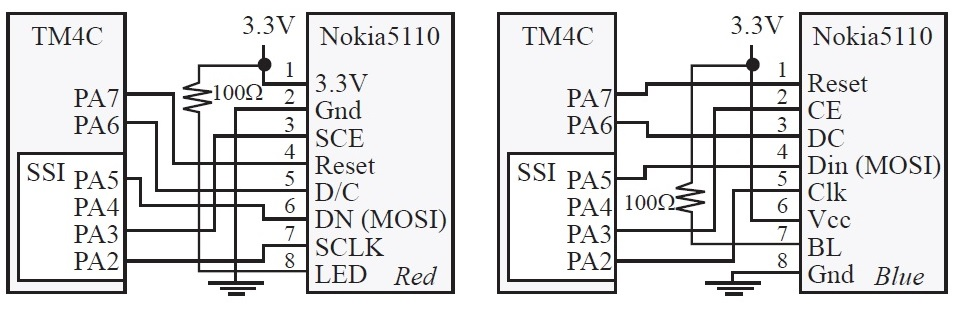Figure 17.8. Nokia 5110 interface to a TM4C123.

The matrix ASCII[] contains the pixel image for each character. Notice the 5 by 8 image for ASCII 0x7F is the University of Texas UT symbol. There is one blank line before the 5 by 8 character and one blank line after making each character 7 wide by 8 pixels tall, see Program 17.10.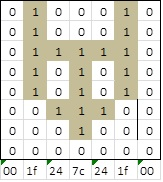Figure 17.9. Nokia 5110 character font is 5 wide by 8-tall pixels.

static const uint8_t ASCII[] = {
{0x00, 0x00, 0x00, 0x00, 0x00} // 20, space
,{0x00, 0x00, 0x5f, 0x00, 0x00} // 21 !
...
,{0x1f, 0x24, 0x7c, 0x24, 0x1f} // 7f UT sign
};

Program 17.10. Nokia 5110 character font is 5 wide by 8-tall pixels.

The same busy-wait synchronization described in the section on ST7735R will work for the Nokia 5100. There is a rich set of graphics functions available for the Nokia 5100, allowing you to create amplitude versus time, or bit-mapped graphics. Refer to the Nokia5100.h header file for more details.

: How does the Nokia 5110 software driver specify color?

## 17.4. Fixed-point Numbers

Video 17. Fixed-point numbers.

: When do we use decimal fixed point rather than binary fixed point?

: We wish to represent the sqrt(2)=1.4142135623730950488016887242097 as a decimal fixed number with a resolution of 0.001. What integer value do we use?

: We wish to represent 0.75 as a binary fixed number with a resolution of 2^-3 (1/8). What integer value do we use?

## 17.5. Numerical Output

Video 17. Converting integers to ASCII characters.

Look up the behavior of the UDIV and MUL assembly instructions. Consider the following assembly program, where R4 is an input parameter.

MOV  R0,#10
UDIV R1,R4,R0
MUL  R2,R1,R0
SUB  R3,R4,R2

For the following three checkpoints, assume R4 initially contains an unsigned integer of value n.

: What is the value of R1 after the UDIV instruction?

: What is the value of R2 after the MUL instruction?

: What is the value of R3 after the SUB instruction?

Video 17. Recursion.

Program 17.11 shows two implementations of factorial. The one on the top uses iteration, and the one on the bottom uses recursion. It is usually the case that a recursive algorithm can be rewritten in iterative form. Nevertheless, sometimes it is more convenient to implement the algorithm in recursive form.

 ; iterative implementation (22 bytes) ; Input: R0 is n ; Output: R0 is Fact(n) ; Assumes: R0 <= 12 (13! overflows) Fact MOV  R1, #1 ; R1 = 1 = total loop CMP  R0, #1 ; is n (R0) <= 1?      BLS  done ; if so, skip to done      MUL  R1, R0, R1 ; total = total*n      SUB  R0, R0, #1 ; n--      B    loop done MOV  R0, R1 ; total = Fact(n)      BX   LR ; recursive implementation (30 bytes) ; Input: R0 is n ; Output: R0 is Fact(n) ; Assumes: R0 <= 12 (13! overflows) Fact CMP  R0, #1 ; is n (R0) <= 1?      BLS  endcase ; if so, to endcase      PUSH {R0, LR} ; save R0 and LR      SUB  R0, R0, #1 ; n--      BL   Fact ; R0 = Fact(n-1)      POP  {R1, LR} ; restore R1, LR      MUL  R0, R0, R1 ; R0 = n*Fact(n-1)           BX   LR ; normal return endcase      MOV  R0, #1 ; R0 = 1      BX   LR ; end case return // iterative implementation // Assumes: n <= 12 uint32_t Fact(uint32_t n){ uint32_t r;   r = 1;   for(; n>1; n--){     r = r*n;   }   return r; } // recursive implementation // Assumes: n <= 12 uint32_t Fact(uint32_t n){   if(n <= 1){ // end condition     return 1;   }   return n*Fact(n-1); // recursion }

Program 17.11. Interative and recursive solutions to factorial.

## EE319K Lab 7 videos

### Educational Objectives of Lab 7

• Variables
— Scope vs persistence
— Local variables on the stack, stack frame
• SPI serial interface to LCD
— Device driver
— Busy-wait synchronization
— Serial transmission
• Fixed-point numbers
— Value = Integer*Constant
• Number to ASCII conversion
• Recursion

### Lab7_Introduction

In this video we review the software starter project and overview the design steps for implementing Lab 7.

### Lab7_ST7735R

We begin Lab 7 by running the existing project ST7735_4C123. This allows us to explore the features of the LCD as well as test hardware connections.

### Lab7_Nokia

If you cannot find a ST7735R LCD, you can complete Labs 7,8, and 10 with the Nokia5110 LCD. Nokia 5110 is available on Amazon. If you complete Lab 7 on the Nokia 5110, plan on using it also for Labs 8 and 10. Download EE319K starter projects for the Nokia 5110. Unzip this file and place the four projects in the same place as your other EE319K projects.

### Lab7_Testing

Once you implement the two functions writecommand and write data in LCD.s, you can test these two function in the simulator or on the real board.

### Lab7_Demo

Demonstration of Lab 7 final solution

JV will pretend to be a student, TA will be a TA, we will tape both JV screen and TA voice to demonstrate how the lab checkout will flow

Reprinted with approval from Embedded Systems: Introduction to ARM Cortex-M Microcontrollers, 2020, ISBN: 978-1477508992, http://users.ece.utexas.edu/~valvano/arm/outline1.htm

and from Embedded Systems: Real-Time Interfacing to ARM® Cortex™-M Microcontrollers, 2020, ISBN: 978-1463590154, http://users.ece.utexas.edu/~valvano/arm/outline.htm